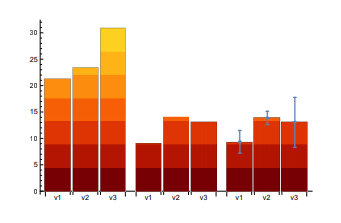Prediction of financial crashes in a complex financial network is known to be an NP-hard problem, i.e., a problem which cannot be solved efficiently with a classical computer. We experimentally explore a novel approach to this problem by using a D-Wave quantum computer to obtain financial equilibrium more efficiently. To be specific, the equilibrium condition of a nonlinear financial model is embedded into a higher-order unconstrained binary optimization (HUBO) problem, which is then transformed to a spin-1/2 Hamiltonian with at most two-qubit interactions.

The problem is thus equivalent to finding the ground state of an interacting spin Hamiltonian, which can be approximated with a quantum annealer. Our experiment paves the way to study quantitative macroeconomics, enlarging the number of problems that can be handled by current quantum computers.

Read the whole article on arVix